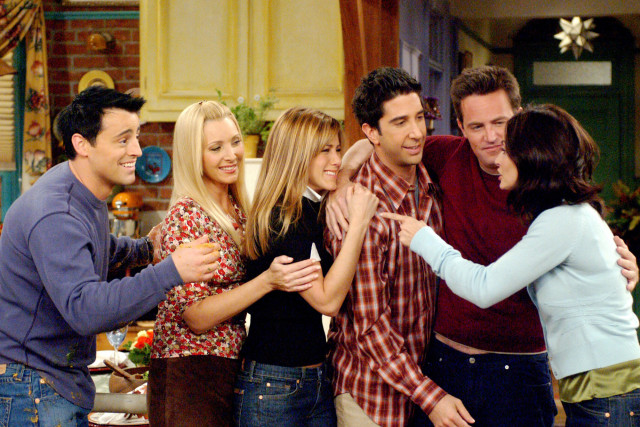# 10 More Life Pro TipsOkay, bear with me, this involves some math:

The Fibonacci Sequence, for those who don’t know, is this: 1, 1, 2, 3, 5, 8, 13, 21, 34, 55, 89, 144, …. Starting with 1, the two neighboring numbers added up equal the next number. One interesting aspect of these numbers is that their ratios all approach being what is called the golden ratio, 1.618….

So, for example, 3/2 is 1.5, 5/3 is 1.666, 8/5 is 1.6, 13/8 is 1.625, 21/13 is 1.615, 34/21 is 1.619, etc.

So what does this have to do with kilometres to miles? Well, the ratio of km to mi is 1.609 to 1. Since 1.609 is so close to the golden ratio, you can use the Fibonacci numbers to convert between kilometres and miles! For example, 5 km is quite close to 3 miles (3.1 mi). 8 km is almost exactly 5 miles, 13 km is 8 miles, 21 km is 13 miles, etc! I think this is awesome.